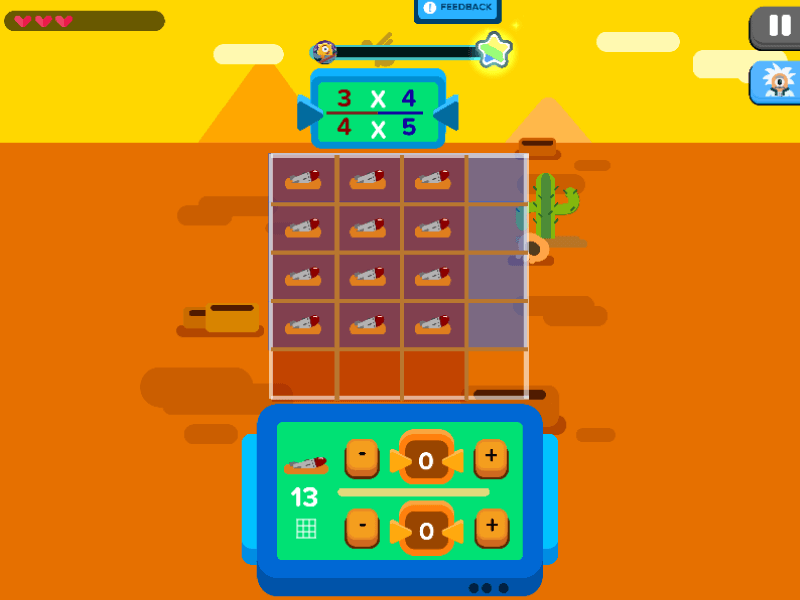## Math Fraction Game : Mine Detector### Mine Detector

In this math fraction game, the city council has developed a new plan to transform an abandoned desert into a picturesque garden for communal harvesting and family gatherings. However, researchers have found landmines hiding under the ground of this abandoned space. The city council requests for your assistance. They need you to use your mathematical expertise to help remove these mines.

In order to get started, gear up and study the two fractions provided on the top of the screen. To remove the landmines, you need to use the grid system to multiply fractions with fractions. This helps identify the exact location of each mine for safe removal from the ground.

After you have studied the two fractions, click on each of them to shift them to the equation box. As you click on each fraction to move them into the equation box, a grid will be built based on the individual fractions and parts. This will reveal an overlapping section of boxes when both fractions are combined, and this will be where the landmines lie!

Now that you have found the mines, you have triggered a timer on them! This means you are only given a very short amount of time to solve the equation and stop the mines from detonating.

To get the answer for the numerator (the top number), count the number of boxes that have mines. To get the answer for the denominator (the bottom number), count the total boxes including the ones with the mines. The right answer will defuse the mines, but the wrong one will detonate them. So count carefully!

Mine Detector is a math fraction game that shows you how to have fun with fractions. A fraction represents a broken part of a whole number. Fractions consist of two parts: the numerator is the name of the number that is above the line, and the denominator is the name of the number below the line. While the numerator signifies a number of equal parts, the denominator tells us how many of those parts make up a whole unit.

This speedy game is an exciting way to practice fractions. It combines basic multiplication with a grid system to further enhance and encourage the understanding of fractions in multiplication.